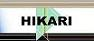| Home | Journals | Books | Paper submission | About us and our mission | News | Contact us |Site Search:

## Online Edition, Vol. 5, 2011, no. 45-48

K.R. Kazmi, S.A. Alvi
Existence and iterative approximation of solutions of multi-valued implicit variational-like inclusions: A strongly P-\eta-proximal mapping approach
Int. Journal of Math. Analysis, Vol. 5, 2011, no. 45-48, 2203-2216.

M. Nakai
Analysis of imputation methods for missing data in AR(1) longitudinal dataset
Int. Journal of Math. Analysis, Vol. 5, 2011, no. 45-48, 2217-2227.

E. Pandey, R. K. Mishra, A. Habib, S. P. Pandey
Error estimation for some modified Szasz-Mirakjan-beta operators
Int. Journal of Math. Analysis, Vol. 5, 2011, no. 45-48, 2229-2235.

K. Kannan, K. Chandrasekhara Rao
Some properties of \tau_1\tau_2-s*g locally closed sets
Int. Journal of Math. Analysis, Vol. 5, 2011, no. 45-48, 3337-2249.

K. Prudhvi, M. Rangamma
A unique common fixed point theorem for six non self-maps in metric spaces
Int. Journal of Math. Analysis, Vol. 5, 2011, no. 45-48, 2251-2257.

D. N. Sain, S. N. Mukhopadhyay
On absolutely n-th continuous functions
Int. Journal of Math. Analysis, Vol. 5, 2011, no. 45-48, 2259-2269.

Weimao Qian, Weiming Gong
A equivalent condition for uniform domains
Int. Journal of Math. Analysis, Vol. 5, 2011, no. 45-48, 2271-2274.

H. Mahzoon
A note on classes of analytic functions concerned with uniformly starlike and convex functions
Int. Journal of Math. Analysis, Vol. 5, 2011, no. 45-48, 2275-2283.

B. E. Kanguzhin, D. B. Nurakhmetov
On properties of systems of root functions of well-posed boundary value problems for the second order differential operator
Int. Journal of Math. Analysis, Vol. 5, 2011, no. 45-48, 2285-2294.

Thick sets and dynamics of operators
Int. Journal of Math. Analysis, Vol. 5, 2011, no. 45-48, 2295-2300.

S. Pinjai, K. Mukdasai
Robust exponential stability for uncertain linear non-autonomous systems with discrete and distributed time-varying delays
Int. Journal of Math. Analysis, Vol. 5, 2011, no. 45-48, 2301-2312.

New extensions of Kannan fixed-point theorem on complete metric and generalized metric spaces
Int. Journal of Math. Analysis, Vol. 5, 2011, no. 45-48, 2313-2320.

Desale, B. S. and Qashash, G. A.
Improper partial bilateral generating functions for some orthogonal polynomials
Int. Journal of Math. Analysis, Vol. 5, 2011, no. 45-48, 2321-2328.

Desale, B. S. and Qashash, G. A.
Trilateral generating function for Hermite, Jacobi and Bessel polynomials
Int. Journal of Math. Analysis, Vol. 5, 2011, no. 45-48, 2329-2335.

I. A. Al-Subaihi, "Mohd Taib." Shatnawi, H. I. Siyyam
A ninth-order iterative method free from second derivative for solving nonlinear equations
Int. Journal of Math. Analysis, Vol. 5, 2011, no. 45-48, 2337-2347.

G. Anello, F. Rania
Existence results for nonlinear problems in R^N involving the p-Laplacian
Int. Journal of Math. Analysis, Vol. 5, 2011, no. 45-48, 2349-2359.

Ghanshyam Bhatt
Construction of wavelet frames using lifting like schemes
Int. Journal of Math. Analysis, Vol. 5, 2011, no. 45-48, 2361-2374.

H. Ziat
Ergodic theorem and strong law of large numbers for unbounded and nonconvex random sets
Int. Journal of Math. Analysis, Vol. 5, 2011, no. 45-48, 2375-2393.

C. Bosch, R. Reyes-Heroles
Pareto optimal partitions for a particular welfare function
Int. Journal of Math. Analysis, Vol. 5, 2011, no. 45-48, 2395-2402.

Li Li, Yan Hao, Shin Min Kang
Convergence of perturbed iterative algorithm for generalized quasi-variational inequalities
Int. Journal of Math. Analysis, Vol. 5, 2011, no. 45-48, 2403-2412.

T. Kim, J. Choi, Y. H. Kim
A note on Bernstein polynomials associated with q-Euler numbers and polynomials
Int. Journal of Math. Analysis, Vol. 5, 2011, no. 45-48, 2413-2420.

B. Rahimi, M. Gholami Porshokouhi, M. Rashidi, M. Gholami
An analytic study of the Cauchy problems
Int. Journal of Math. Analysis, Vol. 5, 2011, no. 45-48, 2421-2425.